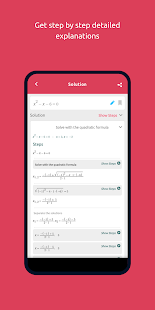Symbolab – Math solver Pro

Your private math tutor, solves any math problem with steps! History! Every problem you solved is saved in history and you can get back to it anytime you want.

Symbolab calculator solves:
Algebra
* Equations
* Inequalities
* System of Equations
* Basic Operations (factor, join, cancel, simplify)
* Partial Fractions
* Polynomial Division
* Percents

Functions & Graphing
* Line equations (line, slope, parallel, perpendicular, midpoint, distance)
* Functions (domain, range, intercepts, asymptotes, extreme points, periodicity, inverse)
* Function arithmetics and compositions

Trigonometry:
* Trig Equations
* Trig identities proving
* Trig function evaluation
* Trig simplification

Calculus
* Limits
* Integrals (definite, indefinite, multiple)
* Derivatives
* Partial derivatives
* Series
* ODE
* Laplace Transform
* Inverse Laplace Transform

Matrices & Vectors
* Matrix Multiply, Power
* Matrix Trace
* Matrix Transpose
* Matrix Determinant
* Matrix Inverse
* Matrix Rank
* Matrix Reduce
* Vector Scalar Multiplication
* Vector Cross Product
* Vector Magnitude
* Vector Angle
* Vector Unit
* Vector Projection
* Vector Scalar Projection
(and more topics are added constantly)
••••